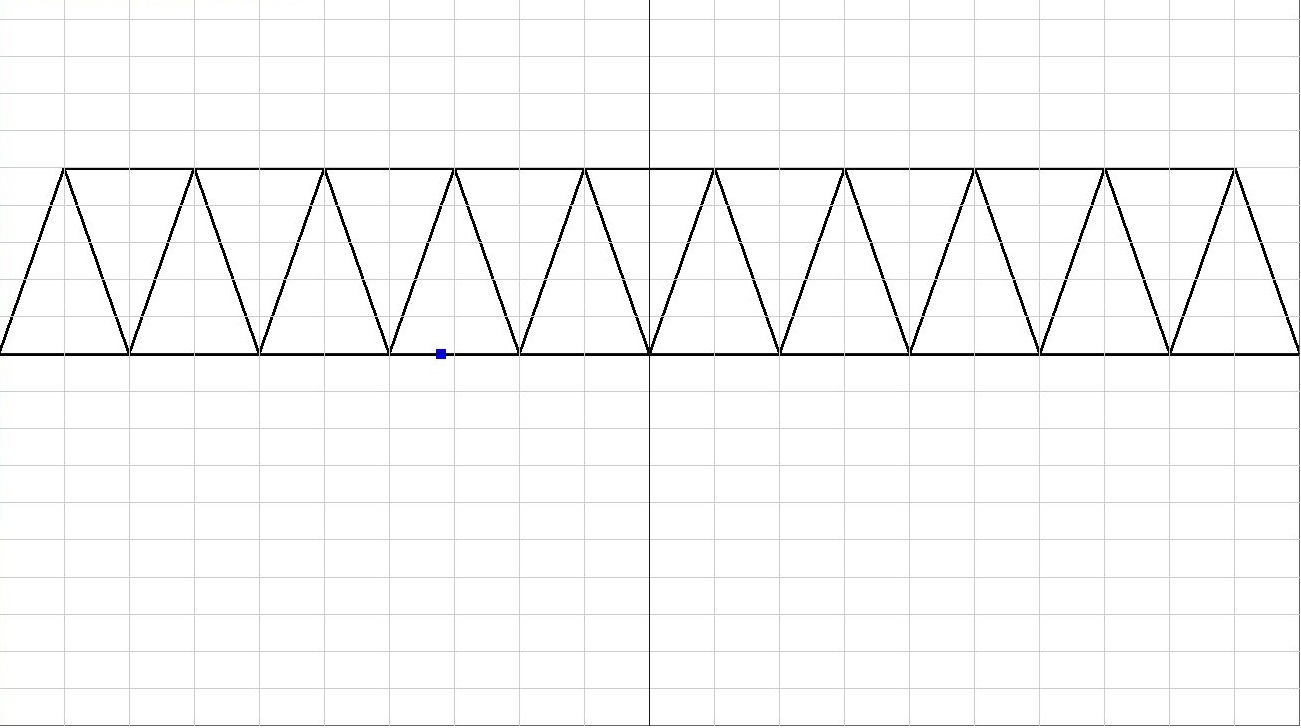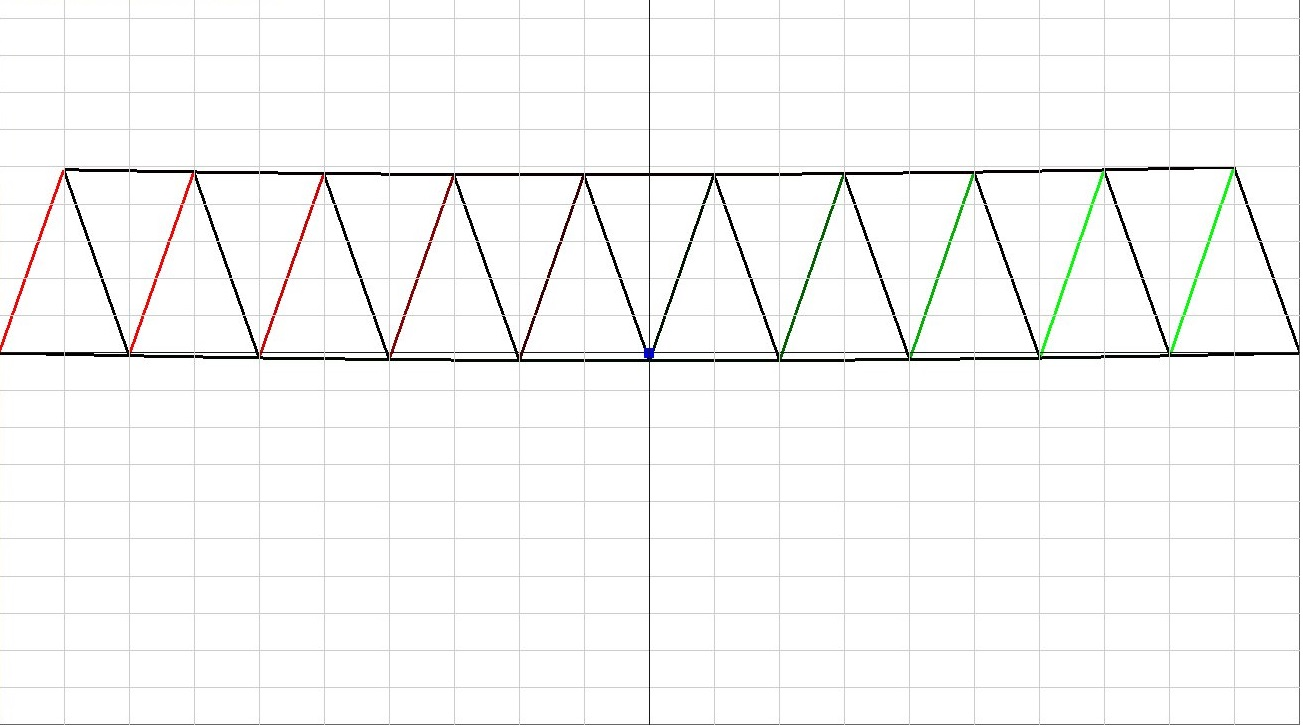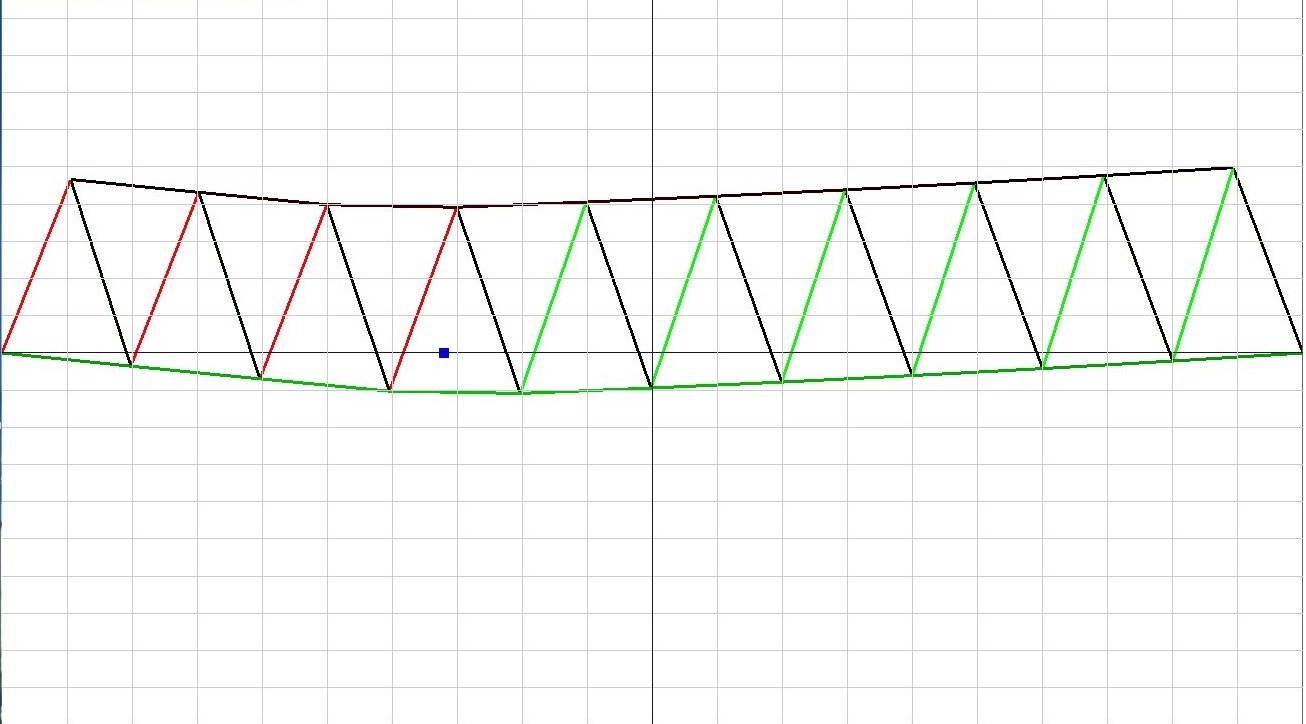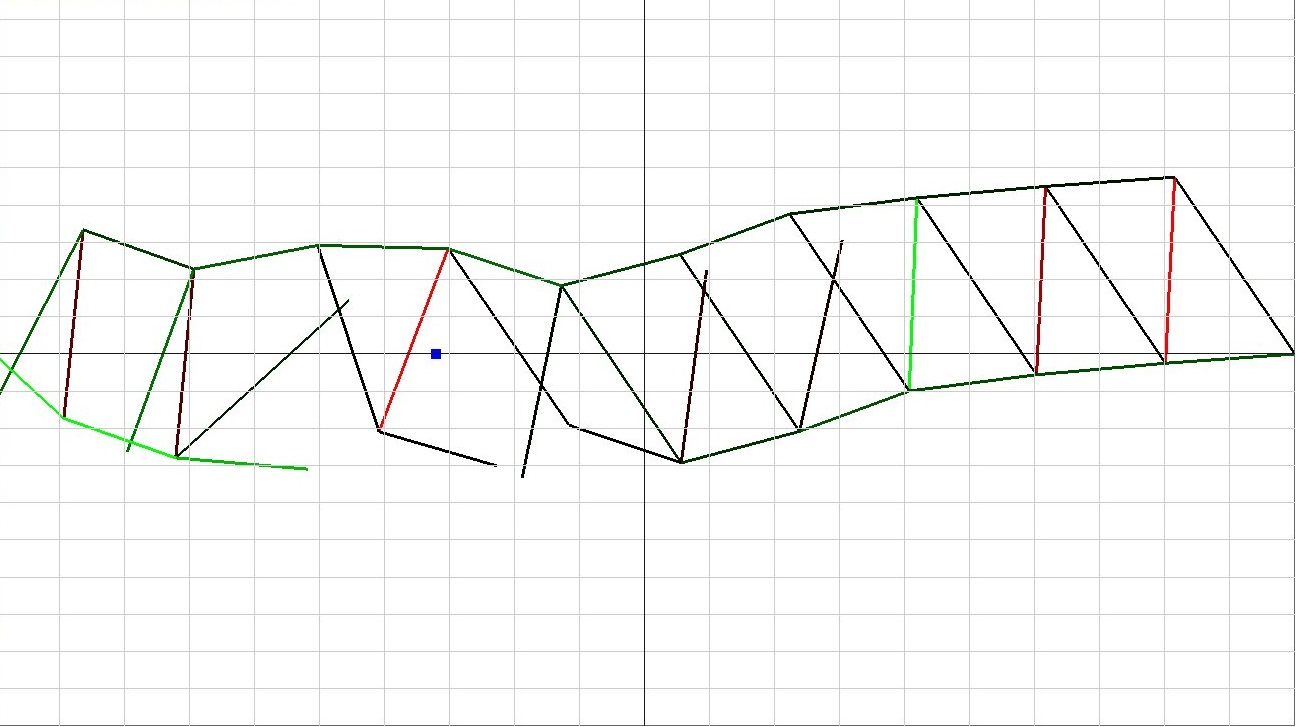# Breaking Bridge

Created by Andrew Wang

## Mass-Spring Physics System

### Springs

Using the Cloth Simulation from Lab 13 and Equations for Springs, I converted it from 3D to 2D and build the bridge beams. Each beam is a "Spring" with K as 1e9.

### Dampening

To prevent the bridge from excessively shaking back and forth. I added dampening so it would not move as much.

### Strain

Using the equation: Strain = (l - L) / L, I could use that to color the beam to determine if it was expanding or compressing.

### Breaking

Using the strain equation on the left, at a certain point of expanding or compressing (Strain greater than 0.1 or less than -0.1). I would break the beam.

## Video and Code Source

You can find the source at my BitBucket
The library I used was Eigen.

## Images#### Initial State

This is the bridge at the start of the program (t = 0).#### No External Force State

This is the bridge as time is on with no forces on the bridge besides the weight of each Beam.#### Strain

This is the bridge with a huge force of pressed on it at a certain point.#### Breaking

With excessive force placed on the bridge, it will break and look similar to this.